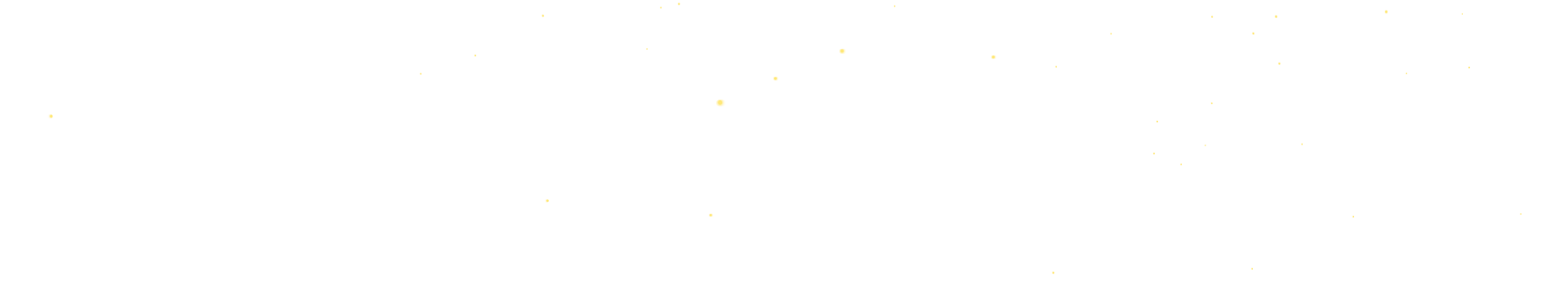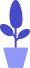# Python Pygame Snake Game Analysis

Estimated reading: 4 minutes 54 views

We learned the new module Pygame earlier, and tried to use it to draw some graphics and write simple animations. In this tutorial, we’ll use Pygame’s GUI to create a classic game called Snake. The interface where the game starts running is shown in Figure 1.figure 1

## program analysis

Snake is a classic puzzle game, available in various versions for PC and mobile, which is simple and playable. Players control the direction of the snake through the up, down, left, and right keys to find food. Each time they eat food, they can get a certain amount of points, and the snake’s body will grow longer and longer. As the snake’s body gets longer, the game becomes more difficult. When the snake touches the surrounding walls, or touches a certain part of its own body, the game is over.

Let’s take a look at the main idea of ​​​​writing this game in Python, and the complete code is given at the end.

#### map

We regard the entire game interface as consisting of many small squares, each of which represents a unit. In this way, the game interface is composed of several small squares to form a map, and each position on the map can be represented as an integer multiple of the small squares, as shown in Figure 2.figure 2
The length of the greedy snake is also represented by this small square. Every time it eats food, the length of the snake’s body will increase by one unit.

#### Program interface

This is a complete game, so we designed a total of 3 interfaces for it, in addition to the game interface, it also includes the game start interface and the game end interface.

#### custom function

The functions we want to create include: main (main program), startGame (starting the game), runGame (running the game), drawFood (drawing food), drawSnake (drawing snakes), drawScore (drawing scores), moveSnake (moving greedy) Snake), isEattingFood (eat food), isAlive (judging whether the snake is dead), gameOver (game over) and terminate (terminate the game).

#### event

The events we are going to use are keyboard events. Keyboard events are events that occur when the player controls the snake to move. We will listen to keyboard events in the startGame() function and gameOver() function described later, and handle them accordingly according to the event type.

#### sound

After the game starts, we will call the play() method of the Sound object to play the background music.

## import module

First, import all modules used in the program. We want to use Pygame functions, so we need to import the Pygame module. In addition to this, we will also use the sys module and the random module. The sys module is responsible for the interaction between the program and the Python interpreter, and is used to manipulate the Python runtime environment. The program needs to use the exit() function of the sys module to exit the game. The random module is used to generate random numbers.

The statements to import these 3 modules are as follows:

``````import pygame
import random
import sys``````

## Initialize settings

In order to prepare for the game to start and run, the program needs to do some initialization settings, including defining the color and direction variables used by the program, determining the size of the game window and map, and defining some other variables that the game needs to use. Let’s take a look at this part of the initialization code first.

#### define color variable

The colors used in the game mainly include the following. In Chapter 12, we introduced the concept of RGB colors and how to use tuples to set color constants.

``````WHITE = (255, 255, 255)
GREEN = (0, 255, 0)
DARKGREEN = (0, 185, 0)
YELLOW = (255,255,0)``````

#### define direction variable

In order to allow the player to control the direction of the snake, we define 4 direction variables in the program, which correspond to the up, down, left, and right of the player’s control of the snake’s movement.

```UP = 1
DOWN = 2
LEFT = 3
RIGHT = 4```

#### define window size

We need to define a window for the snake game, and let the snake move in this window. We use two variables to define the width and height of the window, which is a rectangular window with a width of 800 pixels and a height of 600 pixels.

``````windowsWidth = 800
windowsHeight = 600``````

#### define map size

We use the variables mapWidth and mapHeight to represent the width and height of the map. It should be noted that the width and height of the map are integer multiples of the base unit cellSize.

``````cellSize = 20 #Define the base unit size
mapWidth = int(windowsWidth / cellSize) #The width of the map
mapHeight = int(windowsHeight / cellSize) #The height of the map``````

#### other variables

The program also uses the following two variables:

``````HEAD = 0 # Snake head subscript
snakeSpeed ​​= 7 #The speed of the snake``````